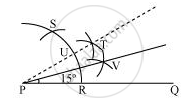# Construct the angles of the following measurement:- 15° - Mathematics

Construct the angles of the following measurement:- 15°

#### Solution

The below given steps will be followed to construct an angle of 15°.

Step I:- Draw the given ray PQ. Taking P as centre and with some radius, draw an arc of a circle which intersects PQ at R.

Step II:- Taking R as centre and with the same radius as before, draw an arc intersecting the previously drawn arc at point S.

Step III:- Taking R and S as centre and with radius more than 1/2RS, draw arcs to intersect each other at T. Join PT.

Step IV:- Let it intersect the arc at U. Taking U and R as centre and with radius more than 1/2RU, draw an arc to intersect each other at V. Join PV which is the required ray making 15° with the given ray PQ.Concept: Basic Constructions
Is there an error in this question or solution?

#### APPEARS IN

NCERT Class 9 Maths
Chapter 11 Constructions
Exercise 11.1 | Q 3.3 | Page 191